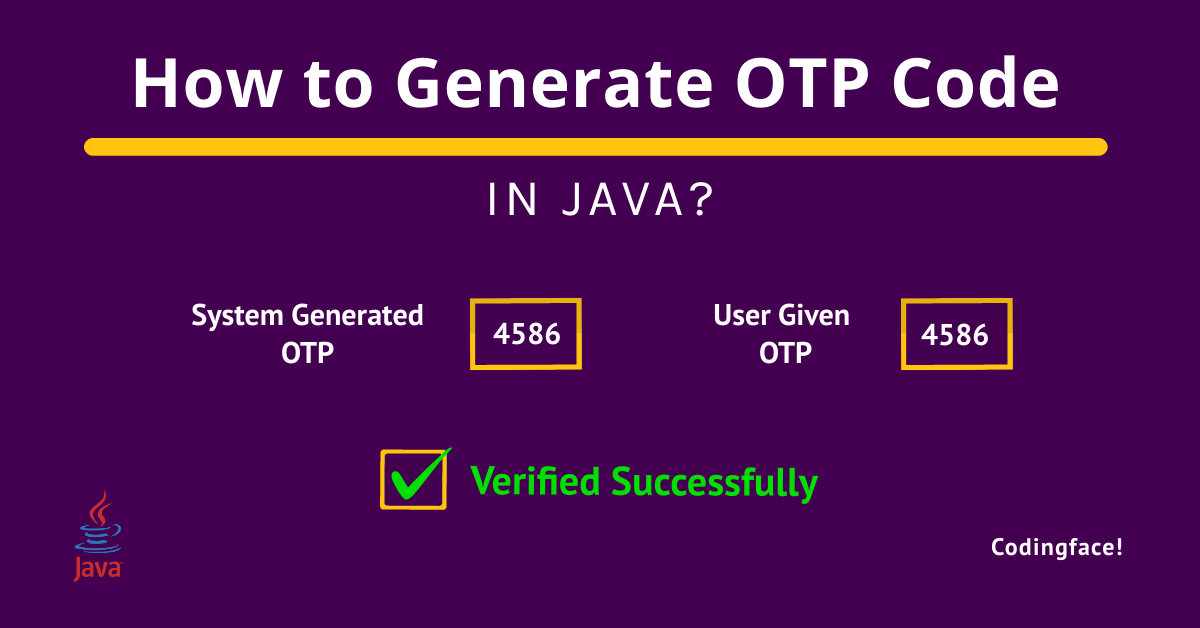# 2 Best Ways to Generate OTP Code in Java

0
30In this tutorial, we will learn how to generate OTP code in Java. We have given 2 approaches to generate OTP randomly by using the random () and concat () methods, and some other logic.

## What is OTP code in Java?

OTP stands for One Time Password, a randomly generated combination of characters or digits which is used to authenticate one user for a one-time transaction or for only one session.

In Java, we can also generate random OTP code by using the simple random () function and some other logic. We will learn how can we achieve that through our simple program.

## Why do we need to generate OTP code in Java?

In most real-time projects, We need to integrate the Authentication functionality into our application, and when the authentication comes in front of the application, OTP plays an important role because of security reasons. As we can use the OTP code for once so it is one of the most secure ways to verify the correct user.

As the OTP is used for one-time validation, is also unique, and important, So we should have the knowledge of generating OTP code in Java while developing any application.

So here, We will learn how to generate OTP code in Java.

## How to generate OTP code in Java?

We can generate OTP code in Java by using a simple random () method, String Concat () method, and some other logic.

First, we will iterate n times to generate random numbers one by one by using the random () method. Suppose we need a 4-digit code for verification, then we will use for loop to iterate 4 times and will generate random numbers one by one 4 times.

Here we will use one empty String to store the generated random numbers one by one by using the concat () method (s = s.concat (Integer.toString (ranNo));). As we can not directly assign one integer to String, so we have used Interger.toString () method to assign the generated random number to String s. Once the above iteration will be completed, we will also get our OTP code.

Let’s see the complete program to understand the above logic.

``````package programs;

import java.util.Random;

public class OTPGenerationCodeInJava

String systemOTP, userOTP;
static String s = "";
static int ranNo;

public static String getOTP(int len)

for (int i = 0; i < len; i++)

ranNo = new Random().nextInt(9);
s = s.concat(Integer.toString(ranNo));

return s;

public static void main(String[] args)

System.out.println("System generated OTP is " + getOTP(4));

```Code language: Java (java)```

### Output

``System generated OTP is 3220``

In the above program, we have seen how can we generate OTP code in Java. In addition to that if you want to verify the generated OTP code that the user given OTP code is the same as the system generated OTP code or notthen you can also follow the below program to understand how to do the verification of OTP code in Java.

Let’s see the below program for the generation and verification of OTP code in Java.

## How to generate and verify OTP code in Java?

``````package programs;

import java.util.Random;
import java.util.Scanner;

public class OTPGenerationCodeInJava

String systemOTP, userOTP, s = "";
int ranNo;

public void getOTP(int len)

for (int i = 0; i < len; i++)

ranNo = new Random().nextInt(9);
s = s.concat(Integer.toString(ranNo));

systemOTP = s;
System.out.println("System generated OTP is " + systemOTP);
Scanner sc = new Scanner(System.in);

userOTP = sc.nextLine();
if (systemOTP.equals(userOTP))
System.out.println("OTP verified successfully");
else

public static void main(String[] args)
OTPGenerationCodeInJava otp = new OTPGenerationCodeInJava();

otp.getOTP(4);

```Code language: Java (java)```

### Output

``````System generated OTP is 7514
OTP verified successfully``````

## Conclusion

In this tutorial we have learned how to generate OTP code in Java and also in the second program, we have learned about the generation and verification of OTP code in Java by using simple random () and concat () methods.

You can use the first program if you just want to generate the OTP code and use the second program if you want both generation and verification of the OTP code. Here we have given the example for the generation of 4 digit OTP code. If you want to create more digit OTP codes, then you can just pass that number of digits you want through the getOTP () method from the main () function (otp.getOTP (4)).

We hope, you can be able to achieve your requirement and like our tutorial. If our tutorial helped you or if you liked our tutorial, then please share it with others.

Thanks & Happy Learning!

## Recommended Articles

1. How To Extend Multiple Class In Java – Complete Guide 2022
2. Best 2 Ways To Give Tab Space In Java
3. Best 2 Ways To Find GCD Of Two Numbers Using Constructor In Java
4. How To Find Multiples Of A Float Value In Java?
5. How To Find Power Of A Number In Java – 3 Simple Ways

## Other Queries We have Covered

• OTP generation and validation in java.
• One-time password generator code in java.
• How to verify system generated and user-provided OTP code in Java?

## FAQs

### What is the OTP code?

OTP stands for One Time Password, a randomly generated combination of characters or digits which is used to authenticate one user for a one-time transaction or for only one session.

### How to generate 6-digit OTP in Java?

You can use the same above program to generate 6-digit or any number of digit OTP in Java. You just need to pass 6 as an argument while calling the getOTP () method (otp.getOTP (4)).

### Which predefined method can we use to generate OTP code in Java?

We can use the random () method to generate OTP code in Java.

Source